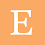Maths Class 10 Chapter 8: Introduction to Trigonometry

# Maths Class 10 Chapter 8: Introduction to Trigonometry

Important Concepts and Formulas

1.      There are 6 trigonometric ratios, i.e., sin, cos, tan, cot, sec and cosec.

2.      In right-angled triangle ABC, right-angled at B,3.      Six trigonometric ratios are related to each other as follows:

4.      If one of the trigonometric ratios of an acute angle is known, the remaining trigonometric ratios of the angle can be easily determined.

5.      The values of trigonometric ratios for some specific angles such as 0°, 30°, 45°, 60° and 90° can be determined easily.

6.      The values of sin A or cos A never exceeds 1, whereas the value of sec A or cosec A is always greater than or equal to 1.

7.      Trigonometric ratios of complementary angles are as follows:

sin (90° A) = cos A ;             cos (90° A) = sin A
tan (90° A) = cot A ;             cot (90° A) = tan A
sec (90° A) = cosec A ;         cosec (90° A) = sec A

8.      These are the trigonometric identities:

sin2 A + cos2 A = 1 ;
sec2 A – tan2 A = 1 ;   for  0° A ≤ 90°
cosec2 A = 1 + cot2 A ;   for  0° < A ≤ 90°

1.